# On the PSPACE-completeness of Bandaged Puzzles

## Introduction

Bandaged puzzles are often noticeably hard to solve. These are ordinary twisty puzzles which have some of their pieces glued together so that they are inseparable. Some examples of bandaged puzzles are the Bandaged Cube, the Square-1, and the Rotascope puzzle shown below.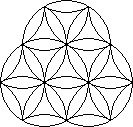→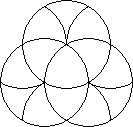The nice mathematical group structure of ordinary twisty puzzles, the symmetry, most of that disappears as moves get blocked and unblocked when you are twisting a bandaged puzzle. There seems to be something inherent to the bandaging that cranks up the difficulty enormously. With a puzzle like the Rubik's Cube, you can increase its size to a 5×5×5, a 7×7×7, or larger, but at a certain point they don't get any harder to solve. It just takes more time. On this page I will show that bandaged puzzles are different. As a puzzle gets bigger, the interaction of the bandages can be made much more complex, so much so that it becomes very hard to figure out if a particular face can even move or not.

## PSPACE and PSPACE-complete

In the theory of computation there are many different types of complexity, and PSPACE is one such type. It is the set of all decision problems that can be solved by a Turing machine using an amount of space that depends polynomially on the size of the problem. This is a complicated definition, so let's break that down a bit.

A decision problem is a question about some type of object (usually a number) with a yes or no answer. For example, with positive numbers you can ask "Is this a prime number?". Or with Rubik's Cube positions you could ask "Is this position solvable in 18 moves?". Generally though, decision problems are about something dependent on positive integers, so that you can examine how much more difficult the problem becomes as the numbers get larger.

If you have an instance of a (decision) problem, its size is the number of digits or bits in the input. This is similar to how in cryptography, the size of encryption keys is their length in bits. The complexity of encryption algorithms is always measured in the length of their keys. Usually this means that if you increment the key size, the difficulty of cracking it approximately doubles. The difficulty is exponential in the size of the input, i.e. approximately proportional to 2n where n is the input size. Many other problems are not exponential. For example, adding or subtracting two numbers of size n takes an amount of time proportional to n. Multiplying two numbers of size n in the normal longhand way takes a time proportional to n2 because that is the number of pairs of digits you have to multiply together. The difficulty of these tasks is polynomial in the size of the input, because it is approximately proportional to some power of n.

A Turing Machine is a very simplistic theoretical computer, with an unlimited amount of memory. It has been proven to be equivalent to any ordinary computer (with enough memory) in what it can calculate, but as it is so stripped down, it is easier to prove things about a Turing machine than about a more general computer.

So, putting this all together, PSPACE is the set of all yes/no problems that a computer can calculate, for which the amount of memory needed depends at most polynomially on the size of the problem. This is clearly a huge set, as there are many problems that don't take much memory to analyse. It also includes some really hard problems. For example, the question "Is there a counterexample to Goldbach's conjecture with at most n digits?" is in PSPACE, since the memory you need to do the arithmetic is proportional to (some power of) n.

There are certain types of PSPACE problems that are so generic, that any other kind of PSPACE problem is essentially a special case of it. These problems are called PSPACE-complete. If you were always able to solve any instance of some type of PSPACE-complete problem quickly compared to the size of the input, then any normal PSPACE problem can also be solved quickly because you can transform it into an instance of that type of PSPACE-complete problem (without making the input too much larger). A type of problem that is PSPACE-complete is therefore at least as hard as any other type of PSPACE problem.

## Boolean Algebra

Boolean algebra, named after the logician George Boole, is a type of algebra operating on variables that don't have numbers as their values, but instead have only the values true or false. Instead of the operations of addition, multiplication, and negation, we have the operations AND, OR, and NOT. The boolean operations AND and OR both operate on two boolean values, to result in a boolean value just like addition and multiplication, while the NOT operation is applied to only one boolean and results in another boolean value, similar to negation.

The AND operation results in a true value if both the operands are true, and results in a false value otherwise. For example, "My Rubik's cube has 12 edge pieces and has 8 corner pieces" is true, because both parts of that statement are true, whereas "My Rubik's cube has 12 edge pieces and has 10 corner pieces" is a false statement because its second part is false. This is fairly logical and intuitive, but it should be pointed out that the word 'and' in the English language is used in many other ways that do not have anything to do with the truth or falsity of the two statements it connects (for example in "I went on a skiing holiday and broke my leg" it gives a temporal connection and even implies a causal connection between the two parts).

The OR operation results in a true value if either or both of the operands are true, and results in a false value only when both operands are false. For example, "My Rubik's cube is solved, or it takes at most 20 moves to solve it" is true regardless of whether or not the cube actually is solved, because the second part of that statement has been proven to be true. Again however, we have to realise that the word 'or' in the English language does not always have this pure logical meaning. It is often used in ways that imply that only one of the statements can be true, not both ("Do you want tea or coffee?") but in boolean algebra, if both operands are true, then the result of OR is also true.

The NOT operation results in a true value if its operand is false, and vice versa. For example, the statement "It is not the case that my Rubik's cube can be solved in at most 20 moves" is false, because the inner statement "My Rubik's cube can be solved in at most 20 moves" is true.

Here is a truth table for AND, OR, and NOT, showing their results for all possible input values.

aba AND ba OR bNOT a
falsefalsefalsefalsetrue
truefalsetrue
truefalsefalsetruefalse
truetruetrue

This system of algebra has associativity and distributivity laws similar to normal algebra:

• a AND (b AND c) = (a AND b) AND c
• a OR (b OR c) = (a OR b) OR c
• a AND (b OR c) = (a AND b) OR (a AND c)
• a OR (b AND c) = (a OR b) AND (a OR c)

There are also De Morgan's laws which deal with how NOT interacts with AND and OR.

• NOT(a AND b) = NOT(a) OR NOT(b)
• NOT(a OR b) = NOT(a) AND NOT(b)

These two laws allow us to push any NOTs down to the lowest level, so that NOT is only applied to single variables or statements.

George Boole invented this system to formalise propositional logic. This was long before electronic computers came along, but when they did, Boolean algebra suddenly became much more important. A boolean value of true or false corresponds to having a wire with a high or a low voltage. With valves, and later transistors, logic gates could be constructed - small circuits with one or two input wires and one output wire which behave just like AND, OR, or NOT. With such logic gates you can build up circuits that do binary arithmetic, the basis for any modern computer. For example, if you add two bits a and b together, the result is a two-bit answer with high bit a AND b, and low bit (a OR b) AND NOT(a AND b). The arithmetic can be much more complex, for example you could even design a circuit with inputs x, y, z, and n, each being a number using k bits, that has the single bit output which is true when xn+yn-zn=0 and false otherwise.

As you can see from that last example, it can be very difficult to figure out whether a circuit, or the equivalent boolean expression can be made true or false. In that example, the expression can only be made true if there is a counterexample to Fermat's last theorem with numbers of at most k bits. Even small expressions may be hard to figure out. For example, is there a choice of boolean values for the variables a, b, and c such that the expression (a OR b) AND (b OR c) AND ((NOT a) OR (NOT c)) AND ((NOT b) OR c) is true?

Working out such an expression can be hard, but it is always possible to just try all combinations of true/false values. Unfortunately there are 2n combinations for n variables, so this way of solving it is exponential in the size of the input, and that quickly becomes infeasible for larger problems. This problem, the satisfiability problem or SAT, is the first to have been proven to be NP-complete, which means it is the most general of problems in the set of NP problems. The set of NP problems is a subset of the PSPACE problems, and is an extremely important part of the theory of computation.

## First order logic

First-order logic is an extension of propositional logic. The latter deals just with statements that must be true or false, and their combinations. First-order logic allows statements that have an unspecified subject (or several of them), and such a statement is not intrinsically true or false until the subject is declared. For example, "The puzzle is solvable in at most 20 moves" can be true or false, depending on what kind of puzzle the statement refers to.

First-order logic also adds quantifiers 'For all' and 'There exists', which allow you to specify what the subject of the subsequent statement refers to. For example, "For all normal Rubik's Cubes, the puzzle is solvable in at most 20 moves", or "There exists a puzzle that is solvable in at most 20 moves".

We can apply this to boolean expressions as well, to get statements such as "There exists a value for b such that for all values of a, (a OR b) AND (NOT(a) OR b) holds true". This is a true statement, because if we choose b to be true, then the inner expression is always true regardless of the value of a. In fact, any computer program that uses a finite amount of memory can be converted to a statement like this. Furthermore, the amount of memory that the program needs is polynomially related to the number of variables used in the expression. The problem of deciding whether boolean expressions with quantified variables are true or false is called the Quantified Boolean Formula Problem (QBFP), and this is a PSPACE-complete type of problem precisely because it is equivalent to computer programs with bounded memory.

## Bandaged Puzzle Gates

With the bulk of the theory out of the way, we can now finally look at bandaged puzzles. We need to build bandaged puzzles with an arbitrarily large number of faces, so it is best to look at planar puzzles rather than three-dimensional ones, because bandaged circle puzzles such as Rotascope can be extended by adding as many faces as are necessary for our purposes.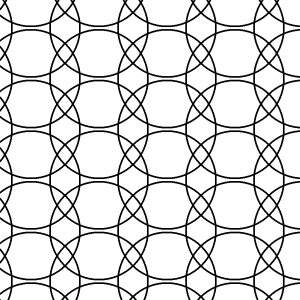Instead of the triangular grid of the Rotascope, I'll choose to use a rectangular grid, as shown in the picture above. Every circle can only turn by 180 degrees, and the circles have a fair amount of overlap so that there are plenty of pieces we can bandage together. With this grid we can make horizontal and vertical 'wires'.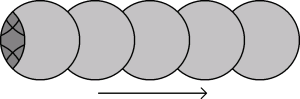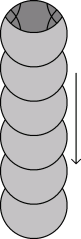The last circle of such a wire can only be moved if all the previous circles have moved first. The small dark grey pieces are like a signal that travels along the wire. We can also turn corners, connecting a vertical to a horizontal wire.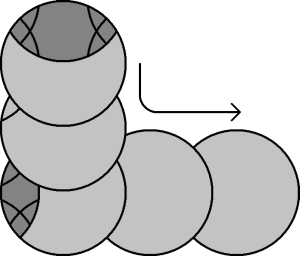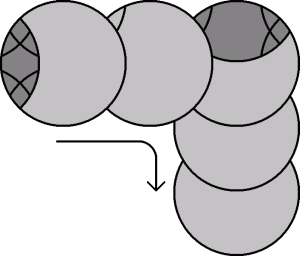We can even allow wires to cross each other, without really interacting.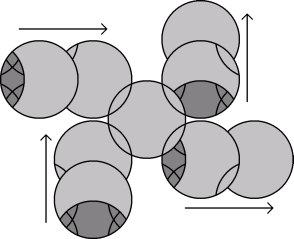A signal can go from the left input to the right output or from the bottom input to the top output, without affecting the flow in the other direction at all. I am assuming however that the centre circle only does half turns, so partial moves are not allowed. Without that assumption a more complicated arrangement is needed for a wire crossing.
More interesting is the fact that we can also build logic gates.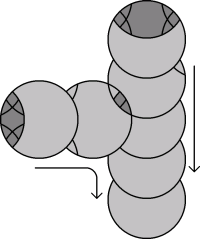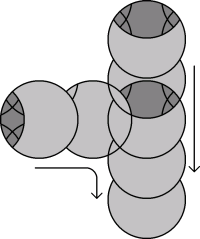You can check that the AND and OR gate shown here act exactly as intended. The output wire of the AND gate shown on the left will only be able to move if both the input wires have been moved all the way to the gate, and the output of the OR gate can get to move if at least one of the input wires has been moved all the way to the gate.

Using these parts as building blocks, we can construct a bandaged puzzle that is the equivalent of any boolean expression that does not have a NOT gate in it. Unfortunately it is not possible to make a NOT gate, because nothing forces you to make a move. It is, however, not necessary to have a NOT gate because we can build a latch.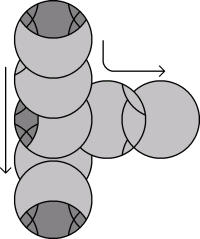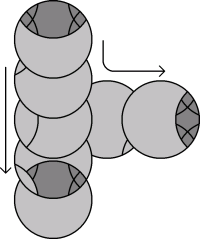A latch is like a switch. It has two outputs, and normally exactly one of them is active. So a latch has an internal state, on or off like a single bit. It has one input, and the latch can only change its state if the input is temporarily made active. The two possible states are shown above. You may notice that the latch is essentially just an OR gate with inputs and outputs reversed. Any variable in a boolean expression can be represented using a latch. One of the two outputs then represents the variable's value v and the other NOT(v). Note however that if the input wire has been activated, then both its outputs are active, so for a latch to properly represent a variable we must ensure that in the end, the input is inactive.

If we reverse an AND gate, we get a splitter.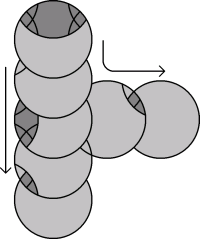A splitter has two outputs and one input, and both outputs become active when the input becomes active, and are inactive when the input is inactive. In essence it splits a wire to allow a signal to go to more than one place.

## Building a Problem

Now let's see how far these building blocks can take us. A SAT problem is the problem of determining if any boolean expression can be made true by a particular choice of variable values. Due to De Morgan's laws we can rewrite any such expression so that any NOTs in the expression only use single variables as their argument. I'll use the example mentioned earlier, the expression (a OR b) AND (b OR c) AND ((NOT a) OR (NOT c)) AND ((NOT b) OR c), and show how to build a bandaged puzzle that is equivalent to the satisfiability problem on that expression.

Firstly, it is easy to build a circuit with 4 OR gates and 3 AND gates that calculates the result of that expression from the inputs a, NOT a, b, NOT b, c, and NOT c.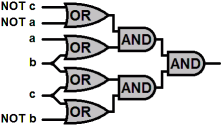Note that two splitters were used as well to deal with any inputs that are used more than once. We have 3 variables in the expression, and we can use three latches to represent them.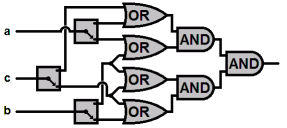Some crossings were needed to match up the latch outputs to the inputs of the boolean expression circuit.

The latches have the problem that if you activate their input, both their outputs can become activated. We need to enforce the fact that we need a solution with all those inputs deactivated so that each latch variable is locked to a properly chosen value. Here we deviate further from normal electronic computing. If one end of a wire needs to be inactive, the other end needs to be active. So let's wire those latch control inputs as well as the output of the boolean expression circuit to the inputs of a set of AND gates. The output of the final AND gate can then only be made active/true, if each of the variable latches have been locked to a particular value, and those values are such that the boolean expression is true.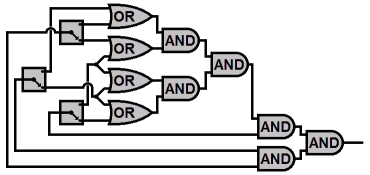This has now become a logic 'circuit' with only one output. If we make this out of bandaged puzzle parts, we get a bandaged puzzle for which the circle at the end of the output wire turns only if the rest of the puzzle is manipulated in a way that is equivalent to finding a solution to the SAT problem we started with.

To make that output circle move, the player has to take the signals up the latch inputs, choose one of the outputs of each latch to propagate a signal through, and make that choice in such a way as to be able to propagate it all the way through the boolean expression part of the circuit, then reverse the latch input signals and take them forward to open the final AND gates, to finally reach the last circle of the output wire.

This example illustrates how we can build a bandaged puzzle to represent any SAT problem, and this already shows that solving bandaged puzzles is at least NP-complete. However it is also possible to represent the quantifiers "For all possible values of variable x such that ..." and "There exists a value for variable x such that ..." by small circuits of these logic gates. I'll skip the details of how to do this, but they can be found in the papers of Robert Hearn (see the references below). This means that any QBFP instance is also equivalent to a bandaged puzzle. The general problem of deciding whether a part of a bandaged circle puzzle can ever be moved is therefore PSPACE-complete.

## References

The main ideas on this page are based on the work on sliding puzzles by Bob Hearn and Erik Demaine. See for example the following paper:
PSPACE-Completeness of Sliding-Block Puzzles and Other Problems through the Non-deterministic Constraint Logic Model of Computation, by Robert A. Hearn and Erik D. Demaine.
My contribution is just to apply those ideas to bandaged circle puzzles by finding the appropriate widgets to act as wires and gates.